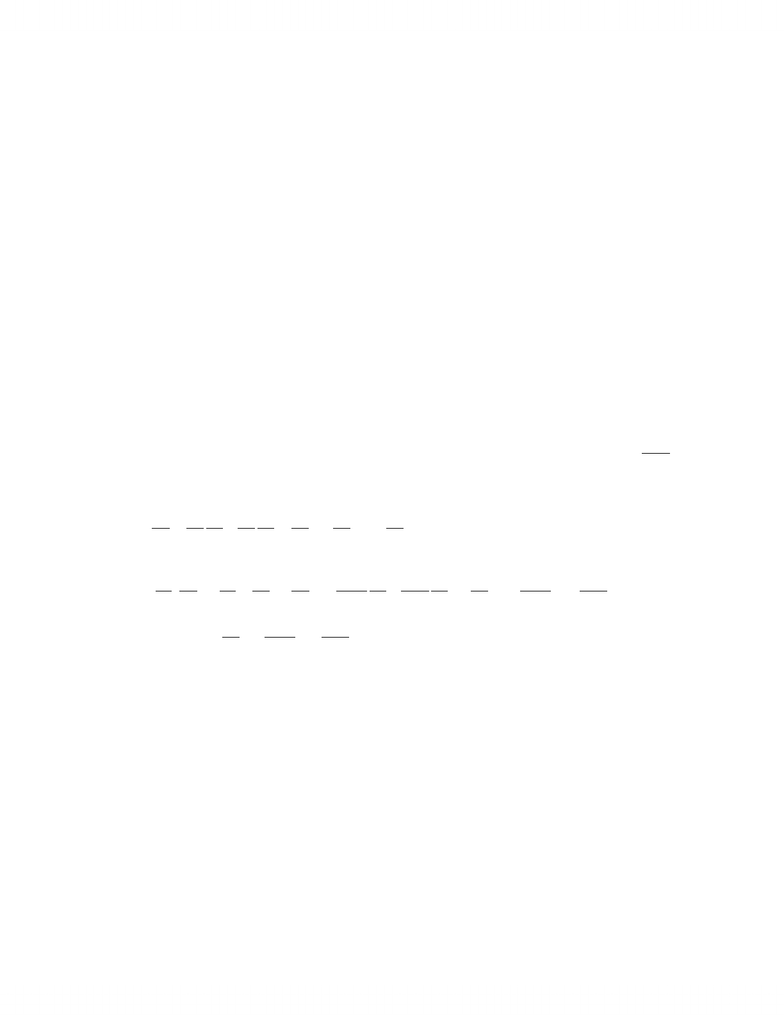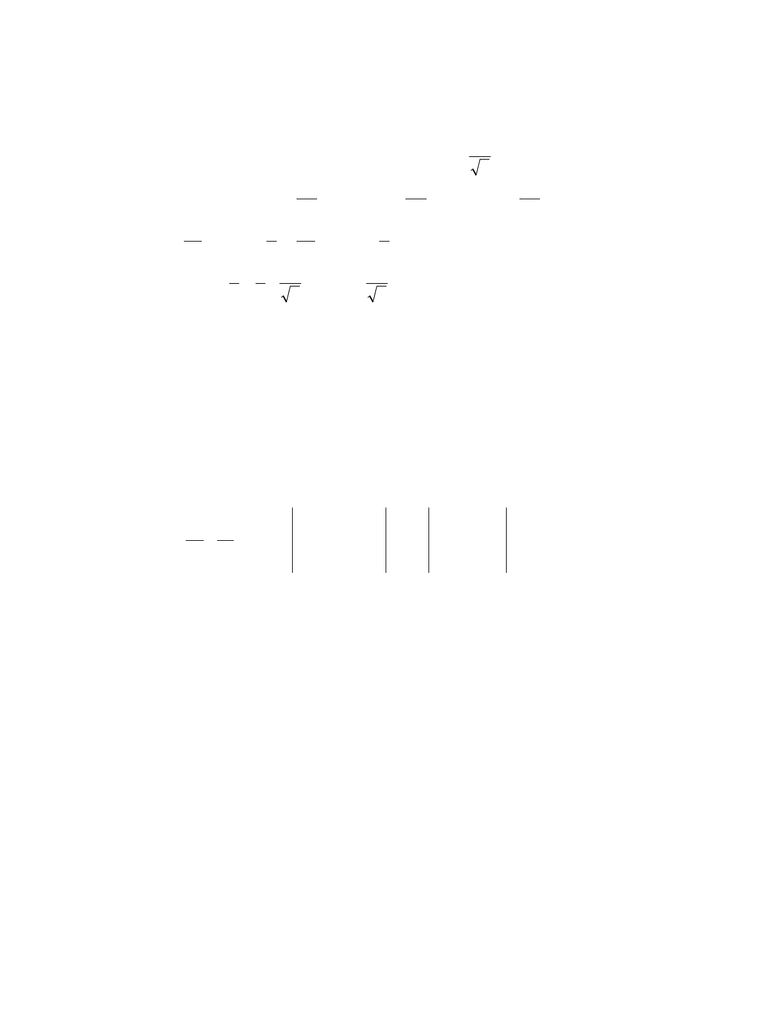Study Guides (390,000)
CA (150,000)
UTSG (10,000)
MAT (1,000)
all (20)

# MAT237Y1 Study Guide - Connected Space, Convex Set, Open Set

This preview shows page 1. to view the full 5 pages of the document.1.(a) [5 marks] Define by . Suppose
32
:RR f),,(),( 2vuvevuvu u++=f22
:RR g
is of class C,
1)1,0()1,1(
=
g, and . Compute
=12
11
)1,1(gD)1,1)(( gf
ο
D.
)1,1()1,0()1,1)(( gfgf DDD =ο
+
=12
11
1
0
12
)1,0(
uv
e
u
u
=12
11
11
01
10
=
03
11
12
(b) [5 marks] Suppose stysxyxfu
=
=
=,2),,( . Assuming f is of class C, find
2
ts
u
2
in
terms of x , y and the partial derivatives of f over x and y .
t
y
y
f
t
x
x
f
t
u
+
=
s
y
f
x
f
+
=0y
f
s
=
=
)( t
u
s
=
)( y
f
s
s
=
+
+
)( 2
22
s
y
y
f
s
x
yx
f
s
y
f=
+
+
2
22
2y
f
st
yx
f
s
y
f
2
22
y
f
y
yx
f
x
y
f
+
+
=
2

Unlock to view full version

Only page 1 are available for preview. Some parts have been intentionally blurred.2. Assume that the equation 0).,(
=
zyxF , where F is of class , defines z implicitly as a
1
C
function of x and y , that is ),( yxgz
=
. Suppose 0)2,2,1(
=
F and )2,3,1()2,2,1(
=
F.
(a) [4 marks] Evaluate in the direction of
)2,1( g
u)1,1(
2
1=
u.
)2,3,1()2,2,1( =F1)2,2,1( =
x
F, 3)2,2,1( =
y
F , 2)2,2,1( =
z
F
Hence 2
1
)2,1( =
x
g , 2
3
)2,1( =
y
g and
2
1
)1,1(
2
1
)
2
3
,
2
1
()2,1( ==g
u
(b) [6 marks] Suppose the surface is parametrized by . S),,(),( 2vuvuevu vu += +
f
Is the surface ),(:
1yxgzS
=
tangent to at the point ? Justify. S)2,2,1(
Remark: Two surfaces and are tangent at the point aS1
S1
SS
if the tangent planes
at a to both surfaces coincide.
By the assumption ( and
1
)2,2,1 SS
)2,2,1( since it corresponds to )1,1(),(
=
vu .
The normal to the surface at the point
1
S)2,2,1(
is n =
1)2,3,1()2,2,1( =
F. The
normal to is S
)1,1(
11
21)1,1)((
+
+
=
×
=
vu
vu
e
ue
vu
kji
ff
n
111
211
=
kji
=)2,3,1(
and it is parallel to . Hence the tangent planes coincide and the surfaces are tangent to
1
n
each other at the point . )2,2,1(
3